×

Search anything:

#### Algorithms Data StructuresGet this book -> Problems on Array: For Interviews and Competitive Programming

Contents:

1. What is a Stack?
2. What is a Linked List?
3. Differences between stack and Linked List.
4. Similarities between stack and Linked List.
5. Implementation.

## What is Stack?

A stack is a linear data structure in which both insertion and deletion operation occurs at one end. It is based on LIFO (last-in-first-out) principle in which both insertion and deletion takes place from one end only. So, stack is basically a container which is closed from one end. In case of an array, we can access any element of an array at any time, whereas in a stack, we can only access the elements of the stack in the sequential manner. In stack, we can only insert elements of same data type.

Operations Performed on a Stack:

1. push(x) : It is used to insert an element to the top of the stack.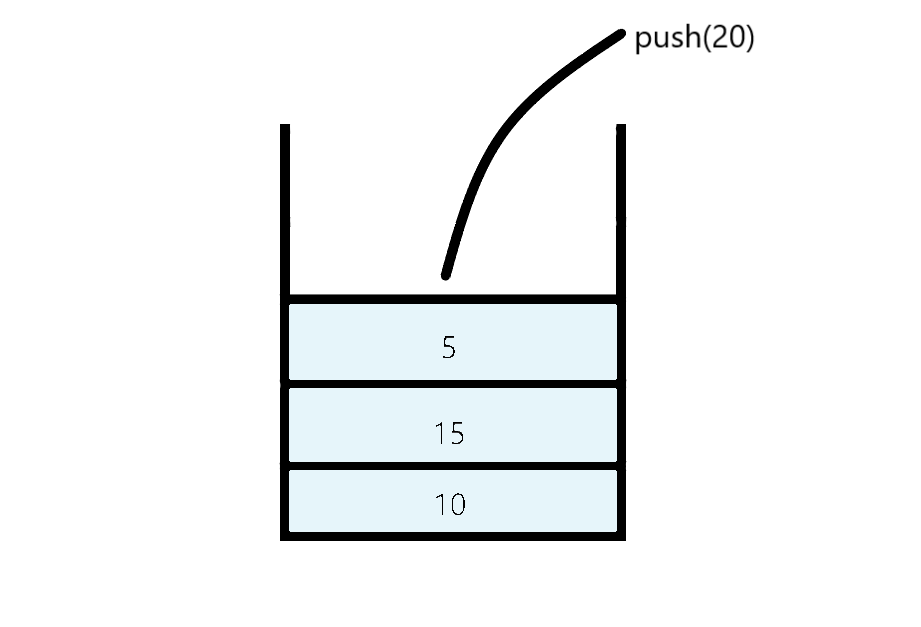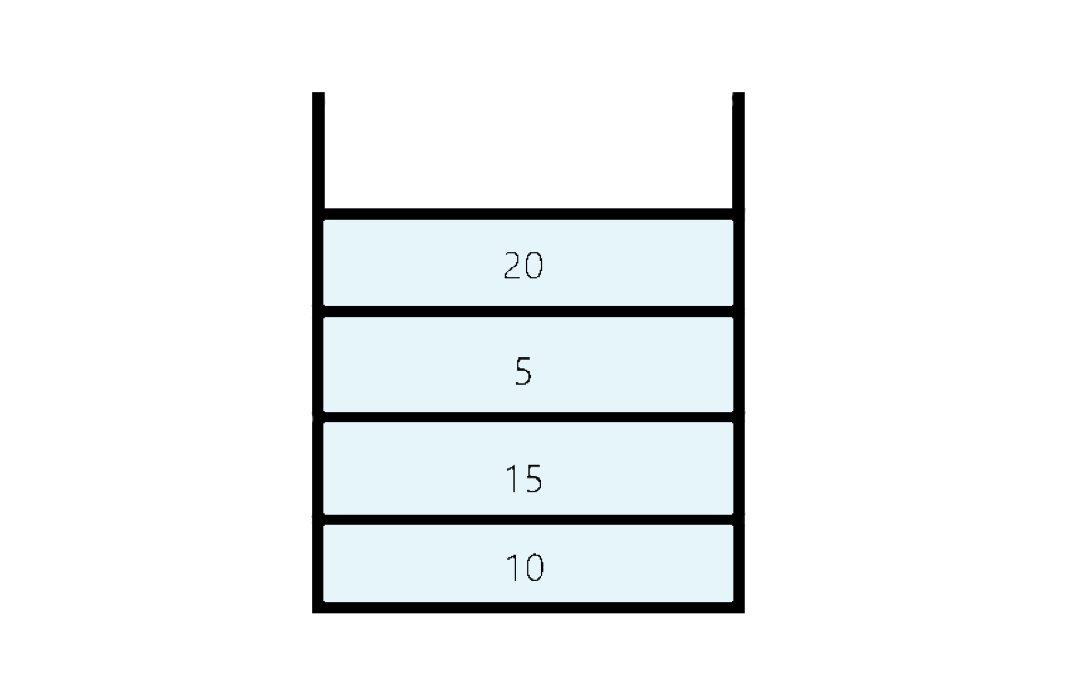2. pop() : It removes the element from the top and returns it value.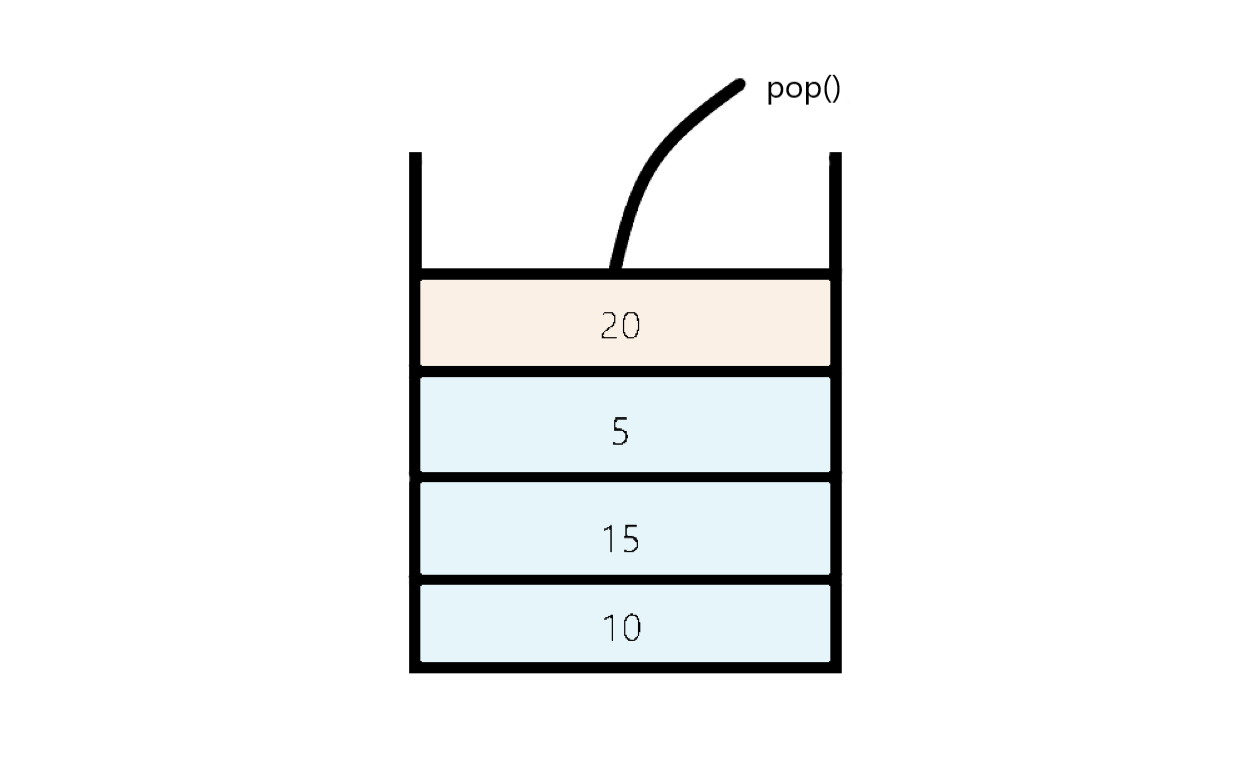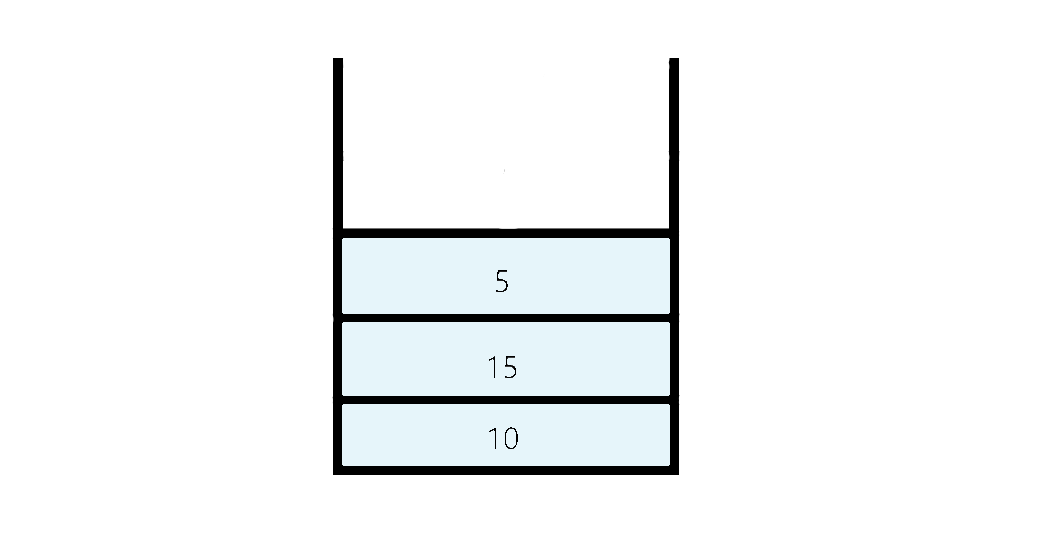3. peek() : It returns the element present at the top.

4. size() : It returns the current size of the size.

5. isEmpty() : Returns true if stack is empty i.e, if there is no elements present in the stack.

Linked List is a linear data structure which store collection of objects known as nodes. These nodes are stored randomly in the memory. The node class contains two fields:

• Data field which store the data of that particular node.
• Reference pointer which stores the address of the next node.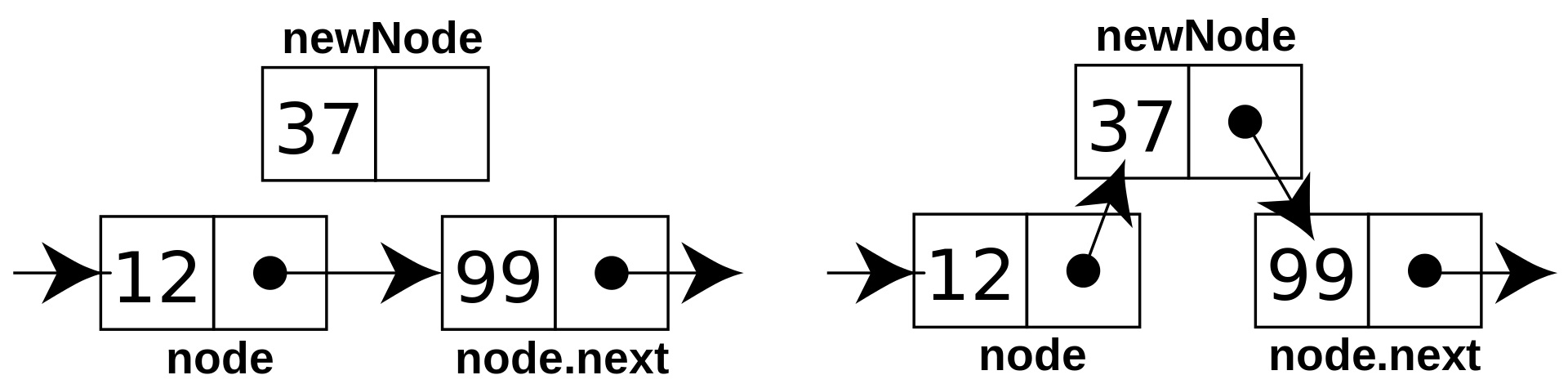We define two pointers head and tail which points to the first node and last node respectively. The last node of the linked list points to null. The list is not required to be contiguously present in the memory. The node can reside any where in the memory and linked together to make a list. This achieves optimized utilization of space.

There are three types of Linked List:
1.Singly Linked List: In singly Linked List each node stores the data and the reference to the next node. It forms a chain like structure. We can Insert, Delete and traverse through a singly Linked List.
2.Doubly Linked List: In doubly Linked List, each node store the data of that node and reference to the next node as well as the previous node. These two reference allows forward as well as backward traversal in the Linked List. Similar to Singly Linked List we can Insert, Delete and Traverse through a Doubly Linked List.
3.Circular Linked List: It is similar to the above Linked List but the only difference is that the last node in the circular Linked List points to the first node, thus forming a circular chain like structure.

## Differences between stack and Linked List.

1. A stack is an abstract data type which is basically a collection of elements like a pile of books. There are basically with two principal operations in stack, which are known as push and pop.
Where as, a linked list is a linear collection of data elements knows as nodes where each node consists value of that node and a reference pointer which points to the next node. The order of the nodes is not given by their location in memory. Thus, this is the main difference between stack and linked list.

2. Push, pop and peek are the main operations performed on a stack while insert, delete and traversing are the main operations performed on a linked list.

3. In a stack, we can only access the topmost element of the stack.
Where as, On the other hand in a linked list, if we wants to access a specific element which is present after i elements, then it is necessary to traverse the first i elements from the beginning of the Linked List to access that particular element.

4. A stack works on the principal of LIFO mechanism, in which the last element inserted will be removed first and both insertion and deletion will took place from that one end only.
whereas, in a linked list, the elements connect to each other by references. Hence, this is another difference between stack and linked list.

5. Implementing a stack is less complex as compared to implementing a Linked List. The structure of stack is also less complex as compared to the linked list.

## Similarities between stack and Linked List.

1. Stack and Linked List both are two different linear data structure. we can implement both of these data structures using any programming language.
2. Both of them are flexible in size and can grow according to requirement of input.
3. we can implement both stack and Linked List using an array.

## Implementation.

Here I am going to implement stack and Linked List:

``````import java.io.*;
import java.util.*;

class Node{
int data;
Node next;
Node(int d){
data=d;
next=null;
}
}

class MyStack{
int size;
MyStack(){
size=0;
}

void push(int x){
Node temp=new Node(x);
size++;
}

int pop(){
{
return Integer.MAX_VALUE;
}
size--;
return res;
}

int peek(){
{
return Integer.MAX_VALUE;
}
}

int size(){
return size;
}

boolean isEmpty(){
}
}

class Test{

public static void main (String[] args)
{
MyStack s=new MyStack();
s.push(15);
s.push(10);
s.push(25);
System.out.println(s.pop());
System.out.println(s.size());
System.out.println(s.peek());
System.out.println(s.isEmpty());
}
}
``````

``````import java.io.*;

Node head; // head of list which will point to the first node of the linked list

static class Node {

int data;
Node next;

// Constructor to inatilise the data and next reference of a node.
Node(int d)
{
data = d;
next = null;
}
}

// Method to insert a new node in the Linked List
{

Node node = new Node(data);
}
else {
while (last.next != null) {
last = last.next;
}
last.next = node;
}
return list;
}

{

// Traversing through the LinkedList to print each element of it
while (currNode != null) {
// Printing the data of the current node
System.out.print(currNode.data + " ");

// iterating to the next node
currNode = currNode.next;
}
}

public static void main(String[] args)
{

//Inserting values in the linked list.
list = insert(list, 1);
list = insert(list, 2);
list = insert(list, 3);
list = insert(list, 4);
list = insert(list, 5);
list = insert(list, 6);
list = insert(list, 7);
list = insert(list, 8);

printList(list);
}
}
``````

The main difference in the dynamic implementation of stack vs queue are:

1. In stack we define only one pointer "head" pointing to the top element of the stack, where as in Linked List we also define one pointers "head" which points to the first node of the Linked List.

2. While inserting element in the stack we make the head pointer point to that element that we are inserting where as, in Linked List we only make the head pointer point to that element only if head if null otherwise, we traverse till the last element in the Linked List and points next of that last element to the node that we have to insert.

3. While removing a element from stack we make the head pointer point to the head.next where as, In Linked List if the node that we want to delete is at the position 0 then,In this case, Change the head of the node to the next node of current head. Otherwise if the node is present in the middle or last, except at head then first we find the previous node and change the next of previous node to the next node of current node.

#### Uddeshya Raj

Uddeshya Raj is pursuing B. Tech in Computer Science from Chandigarh University. He is an Algorithm and Data Structure Developer, Intern at OpenGenus.